The OpenFOAM Foundation
Boussinesq< Specie > Class Template Reference

Incompressible gas equation of state using the Boussinesq approximation for the density as a function of temperature only: More...

Inheritance diagram for Boussinesq< Specie >: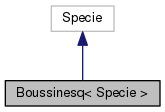[legend]
Collaboration diagram for Boussinesq< Specie >:[legend]

## Public Member Functions

Boussinesq (const Specie &sp, const scalar rho0, const scalar T0, const scalar beta)
Construct from components. More...

Boussinesq (const dictionary &dict)
Construct from dictionary. More...

Boussinesq (const word &name, const Boussinesq &)
Construct as named copy. More...

autoPtr< Boussinesqclone () const
Construct and return a clone. More...

scalar rho (scalar p, scalar T) const
Return density [kg/m^3]. More...

scalar H (const scalar p, const scalar T) const
Return enthalpy departure [J/kg]. More...

scalar Cp (scalar p, scalar T) const
Return Cp departure [J/(kg K]. More...

scalar S (const scalar p, const scalar T) const
Return entropy [J/(kg K)]. More...

scalar psi (scalar p, scalar T) const
Return compressibility rho/p [s^2/m^2]. More...

scalar Z (scalar p, scalar T) const
Return compression factor []. More...

scalar CpMCv (scalar p, scalar T) const
Return (Cp - Cv) [J/(kg K]. More...

void write (Ostream &os) const
Write to Ostream. More...

void operator= (const Boussinesq &)

void operator+= (const Boussinesq &)

void operator*= (const scalar)

## Static Public Member Functions

static autoPtr< BoussinesqNew (const dictionary &dict)

static word typeName ()
Return the instantiated type name. More...

## Static Public Attributes

static const bool incompressible = true
Is the equation of state is incompressible i.e. rho != f(p) More...

static const bool isochoric = false
Is the equation of state is isochoric i.e. rho = const. More...

## Friends

Boussinesq operator+ (const Boussinesq &, const Boussinesq &)

Boussinesq operator* (const scalar s, const Boussinesq &)

Boussinesq operator== (const Boussinesq &, const Boussinesq &)

Ostreamoperator (Ostream &, const Boussinesq &)

## Detailed Description

### template<class Specie> class Foam::Boussinesq< Specie >

Incompressible gas equation of state using the Boussinesq approximation for the density as a function of temperature only:

```    rho = rho0*(1 - beta*(T - T0))
```
Source files

Definition at line 52 of file Boussinesq.H.

## ◆ Boussinesq() [1/3]

 Boussinesq ( const Specie & sp, const scalar rho0, const scalar T0, const scalar beta )
inline

Construct from components.

Definition at line 33 of file BoussinesqI.H.

References Foam::constant::physicoChemical::b, and Foam::name().

Here is the call graph for this function: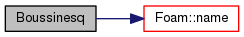## ◆ Boussinesq() [2/3]

 Boussinesq ( const dictionary & dict )

Construct from dictionary.

Definition at line 33 of file Boussinesq.C.

## ◆ Boussinesq() [3/3]

 Boussinesq ( const word & name, const Boussinesq< Specie > & )
inline

Construct as named copy.

## ◆ clone()

 Foam::autoPtr< Foam::Boussinesq< Specie > > clone ( ) const
inline

Construct and return a clone.

Definition at line 60 of file BoussinesqI.H.

References Boussinesq< Specie >::New().

Here is the call graph for this function: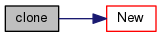## ◆ New()

 Foam::autoPtr< Foam::Boussinesq< Specie > > New ( const dictionary & dict )
inlinestatic

Definition at line 72 of file BoussinesqI.H.

References dict, and Boussinesq< Specie >::rho().

Referenced by Boussinesq< Specie >::clone().

Here is the call graph for this function: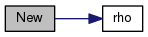Here is the caller graph for this function: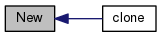## ◆ typeName()

 static word typeName ( )
inlinestatic

Return the instantiated type name.

Definition at line 140 of file Boussinesq.H.

## ◆ rho()

 Foam::scalar rho ( scalar p, scalar T ) const
inline

Return density [kg/m^3].

Definition at line 87 of file BoussinesqI.H.

Referenced by Boussinesq< Specie >::New().

Here is the caller graph for this function: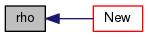## ◆ H()

 Foam::scalar H ( const scalar p, const scalar T ) const
inline

Return enthalpy departure [J/kg].

Definition at line 97 of file BoussinesqI.H.

## ◆ Cp()

 Foam::scalar Cp ( scalar p, scalar T ) const
inline

Return Cp departure [J/(kg K].

Definition at line 104 of file BoussinesqI.H.

References Boussinesq< Specie >::S().

Here is the call graph for this function: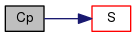## ◆ S()

 Foam::scalar S ( const scalar p, const scalar T ) const
inline

Return entropy [J/(kg K)].

Definition at line 112 of file BoussinesqI.H.

References Boussinesq< Specie >::psi().

Referenced by Boussinesq< Specie >::Cp().

Here is the call graph for this function: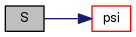Here is the caller graph for this function: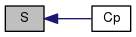## ◆ psi()

 Foam::scalar psi ( scalar p, scalar T ) const
inline

Return compressibility rho/p [s^2/m^2].

Definition at line 123 of file BoussinesqI.H.

References Boussinesq< Specie >::Z().

Referenced by Boussinesq< Specie >::S().

Here is the call graph for this function: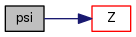Here is the caller graph for this function: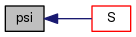## ◆ Z()

 Foam::scalar Z ( scalar p, scalar T ) const
inline

Return compression factor [].

Definition at line 134 of file BoussinesqI.H.

References Boussinesq< Specie >::CpMCv().

Referenced by Boussinesq< Specie >::psi().

Here is the call graph for this function: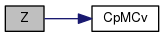Here is the caller graph for this function: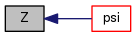## ◆ CpMCv()

 Foam::scalar CpMCv ( scalar p, scalar T ) const
inline

Return (Cp - Cv) [J/(kg K].

Definition at line 145 of file BoussinesqI.H.

References R.

Referenced by Boussinesq< Specie >::Z().

Here is the caller graph for this function: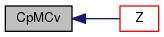## ◆ write()

 void write ( Ostream & os ) const

Write to Ostream.

Definition at line 47 of file Boussinesq.C.

Here is the call graph for this function: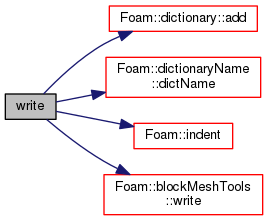## ◆ operator=()

 void operator= ( const Boussinesq< Specie > & )
inline

Definition at line 158 of file BoussinesqI.H.

## ◆ operator+=()

 void operator+= ( const Boussinesq< Specie > & )
inline

Definition at line 172 of file BoussinesqI.H.

References Foam::mag(), Y, Y1, and Y2.

Here is the call graph for this function: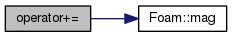## ◆ operator*=()

 void operator*= ( const scalar s )
inline

Definition at line 192 of file BoussinesqI.H.

References Foam::constant::physicoChemical::b, Foam::mag(), s(), Y1, and Y2.

Here is the call graph for this function: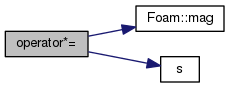## ◆ operator+

 Boussinesq operator+ ( const Boussinesq< Specie > & , const Boussinesq< Specie > & )
friend

## ◆ operator*

 Boussinesq operator* ( const scalar s, const Boussinesq< Specie > & )
friend

## ◆ operator==

 Boussinesq operator== ( const Boussinesq< Specie > & , const Boussinesq< Specie > & )
friend

## ◆ operator

 Ostream& operator ( Ostream & , const Boussinesq< Specie > & )
friend

## ◆ incompressible

 const bool incompressible = true
static

Is the equation of state is incompressible i.e. rho != f(p)

Definition at line 151 of file Boussinesq.H.

## ◆ isochoric

 const bool isochoric = false
static

Is the equation of state is isochoric i.e. rho = const.

Definition at line 154 of file Boussinesq.H.

The documentation for this class was generated from the following files:
• src/thermophysicalModels/specie/equationOfState/Boussinesq/Boussinesq.H
• src/thermophysicalModels/specie/equationOfState/Boussinesq/Boussinesq.C
• src/thermophysicalModels/specie/equationOfState/Boussinesq/BoussinesqI.H